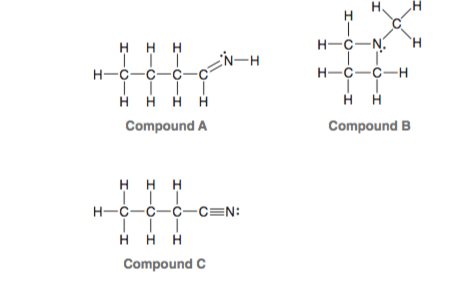Problem: Consider the three compounds shown below and then answer the questions that follow: (b) Which compound contains a nitrogen atom with trigonal pyramidal geometry?

FREE Expert Solution
90% (417 ratings)
Problem Details

Consider the three compounds shown below and then answer the questions that follow:

(b) Which compound contains a nitrogen atom with trigonal pyramidal geometry?What scientific concept do you need to know in order to solve this problem?

Our tutors have indicated that to solve this problem you will need to apply the Molecular Geometry concept. You can view video lessons to learn Molecular Geometry Or if you need more Molecular Geometry practice, you can also practice Molecular Geometry practice problems .

What is the difficulty of this problem?

Our tutors rated the difficulty of Consider the three compounds shown below and then answer the... as low difficulty.

How long does this problem take to solve?

Our expert Organic tutor, Jonathan took 1 minute to solve this problem. You can follow their steps in the video explanation above.

What professor is this problem relevant for?

Based on our data, we think this problem is relevant for Professor Hyvl's class at HAWAII.

What textbook is this problem found in?

Our data indicates that this problem or a close variation was asked in . You can also practice practice problems .